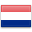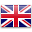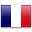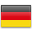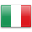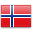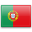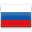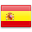# 6094MOR1

• 1ProEXR File Description

=Attributes=
cameraAperture (float): 36.000004
cameraFNumber (float): 8.000000
cameraFarClip (float): 1000000015047466200000000000000.000000
cameraFarRange (float): 1000.000000
cameraFocalLength (float): 18.000002
cameraFov (float): 89.942513
cameraNearClip (float): 0.000000
cameraNearRange (float): 0.000000
cameraProjection (int): 0
cameraTargetDistance (float): 179.491241
cameraTransform (m44f)
channels (chlist)
compression (compression): Zip
dataWindow (box2i): [0, 0, 3999, 2248]
displayWindow (box2i): [0, 0, 3999, 2248]
lineOrder (lineOrder): Increasing Y
name (string): “”
pixelAspectRatio (float): 1.000000
screenWindowCenter (v2f): [0.000000, 0.000000]
screenWindowWidth (float): 1.000000
type (string): “scanlineimage”

=Channels=
A (half)
B (half)
G (half)
R (half)
VRayDenoiser.B (half)
VRayDenoiser.G (half)
VRayDenoiser.R (half)
VRayExtraTex_Oambiental.B (half)
VRayExtraTex_Oambiental.G (half)
VRayExtraTex_Oambiental.R (half)
VRayMtlID.B (half)
VRayMtlID.G (half)
VRayMtlID.R (half)
VRayReflection.B (half)
VRayReflection.G (half)
VRayReflection.R (half)
VRayRefraction.B (half)
VRayRefraction.G (half)
VRayRefraction.R (half)
VRaySelfIllumination.B (half)
VRaySelfIllumination.G (half)
VRaySelfIllumination.R (half)
VRayWireColor.B (half)
VRayWireColor.G (half)
VRayWireColor.R (half)
atmosphere.B (half)
atmosphere.G (half)
atmosphere.R (half)
background.B (half)
background.G (half)
background.R (half)
defocusAmount (half)
diffuseFilter.B (half)
diffuseFilter.G (half)
diffuseFilter.R (half)
globalIllumination.B (half)
globalIllumination.G (half)
globalIllumination.R (half)
lighting.B (half)
lighting.G (half)
lighting.R (half)
noiseLevel (half)
reflectionFilter.B (half)
reflectionFilter.G (half)
reflectionFilter.R (half)
refractionFilter.B (half)
refractionFilter.G (half)
refractionFilter.R (half)
specular.B (half)
specular.G (half)
specular.R (half)
worldNormals.X (half)
worldNormals.Y (half)
worldNormals.Z (half)
worldPositions.X (half)
worldPositions.Y (half)
worldPositions.Z (half)

Compare listings